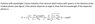# Compton Effect with the electron initially moving

• sciencec

#### sciencec

Homework Statement
A photon with wavelength (lambda) moves towards a free electron which moves with speed u in the direction of the incident photon (see figure). if the photon disperses at angle theta, show that the wavelength of the dispersed photon is: (see picture below)
Relevant Equations
Relativity Formulas, Doppler Effect, etc
Hello! I do not understand how to prove the exercise. I have searched all over but I have found no hints on how to get started. Can anybody help me?

#### Attachments

•BB3700FB-5342-492D-8BF9-B966A0676B8C.jpeg
24.3 KB · Views: 82

What about transforming to and from the rest frame of the electron?

How about starting by writing something down? What two equations do you need and what form do they take?

What about transforming to and from the rest frame of the electron?
that’s what i don’t get. transform what? the frequencies or the velocities?

How about starting by writing something down? What two equations do you need and what form do they take?
I’m not sure what equations I need. I am conflicted between needing the doppler effect formulas (fobs=sqrt((1-u/c)/(1+u/c))fsource) and the compton scattering formula

Start with the standard energy and momentum conservation equations. You asked in #4 what to transform, frequencies or velocities? The answer is both are different in the two frames excepting, of course, the speed of light.

that’s what i don’t get. transform what? the frequencies or the velocities?
Everything! That's what you normally do when you change frames.

Everything! That's what you normally do when you change frames.
So I started with setting the frame S’ as the frame where the electron is at rest and the dispersion angle is theta’. So the velocities in the S’ frame would be: Ux’=Ux-v/1-(Ux(V))/c^2 and so the cos(theta’)=Ux’/c. I then substituted that cos(theta’) into the compton scattering equation. is that correct so far?

So I started with setting the frame S’ as the frame where the electron is at rest and the dispersion angle is theta’. So the velocities in the S’ frame would be: Ux’=Ux-v/1-(Ux(V))/c^2 and so the cos(theta’)=Ux’/c. I then substituted that cos(theta’) into the compton scattering equation. is that correct so far?
Without calculation the velocity of the electron is zero in its rest frame.

Without calculation the velocity of the electron is zero in its rest frame.
I’m still very clueless as to what to do. The teacher has never explained this topic and to add to that, english isn’t even my first language. Could you give me some more hints? This exercise has truly taken too much of my time. I would be very greatful

I’m still very clueless as to what to do. The teacher has never explained this topic and to add to that, english isn’t even my first language. Could you give me some more hints? This exercise has truly taken too much of my time. I would be very greatful
By the way, I greatly appreciate the help and am not looking to have the answer given to me. I want to learn, but sometimes it’s very stressful when there is a due date and the teacher hates helping the students.

I’m still very clueless as to what to do. The teacher has never explained this topic and to add to that, english isn’t even my first language. Could you give me some more hints? This exercise has truly taken too much of my time. I would be very greatful
The first step is to transform the data to the electron rest frame. You must be able to do that as a first step. If you are not able yet to do that then there's not a lot we can do to help.

The first step is to transform the data to the electron rest frame. You must be able to do that as a first step. If you are not able yet to do that then there's not a lot we can do to help.
In the electron rest frame its velocity would be cero and the compton formula would apply as it applies in the case of the electron being at rest

In the electron rest frame its velocity would be cero and the compton formula would apply as it applies in the case of the electron being at rest
Yes, but we need to see your calculations for the energy, wavelength and scattering angle in that frame.

This is an advanced problem, so you need to show some understanding of material at this level.